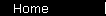### Measurement Converter

=

=

=

=

#### V. Temperature

(Round I - IV to significant digits)

#### The Logic Behind the Units of Measure

 English Metric Length: The small units are based on body measurements: an inch is the width of a man's thumb, a foot is the length of an ill-fitting shoe, a yard is the distance from your nose to your outstretched pinkie... A furlong is apparently a natural length to make a furrow, if you're plowing one behind an ox... A mile is the distance covered in 1000 paces (that is, two-step paces covering about five feet total). Metric lengths are based on the circumference of the Earth: the distance from the poles to the equator is approximately ten thousand kilometers. Area: Mostly obvious: just squares of length units. An acre is one tenth of a square furlong. All obvious: squares of length units. Volume: Mostly obvious: cubes of length units. The gallon is the mysterious unit: it's approximately the volume of eight pounds of water, and also roughly one eighth of a cubic foot... maybe that had something to do with it. (8 is a natural factor to use in volumes, since it's a perfect cube.) All obvious: cubes of length units. (A liter is 1000 cubic centimeters.) (1000 is another natural factor to use in volumes, since it's another perfect cube.) Weight: An (avoirdupois) ounce is approximately the weight of one (fluid) ounce of water. A kilogram is the mass of one liter of water. Temp: Fahrenheit apparently just liked 32o for the freezing point of water and 96o for human body temperature. (96 is 3x32; Fahrenheit wrote that a person with a fever might get as hot as 128o, which is 4x32. The guy thought in terms of 32, possibly because rulers divide inches into 32nds.) Later the scale was recalibrated to make the difference between the boiling point and the freezing point equal an even 180 degrees. Water freezes at 0o C and boils at 100.(Celsius designed his scale upside-down, with the boiling point at zero, which is why it seems more logical to say Centigrade than Celsius.)

 home  |  features  |  diversions  |  glossary  |  calculator  |  about us  |  books